# Transformer

Solve the textbook problems - transformer:

a) N1 = 40, N2 = 80, U2 = 80 V, U1 =?

b) N1 = 400, U1 = 200 V, U2 = 50 V, N2 =?

U1 =  40 V
N22 =  100

### Step-by-step explanation: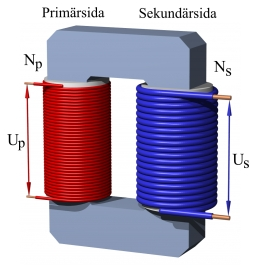Did you find an error or inaccuracy? Feel free to write us. Thank you!Tips to related online calculators
Check out our ratio calculator.

## Related math problems and questions:

• Two resistorsTwo resistors 20 Ω and 60 Ω are connected in series and an external voltage of 400 V is connected to them. What are the electrical voltages on the respective resistors? Please comment!
• TransformerTransformer - U1 = 230 V, N1 = 300, N2 = 1,200, I1 = 4 A. Calculate the transformation ratio, voltage and current in the secondary coil.
• Electric input powerSolve problems related to electric power: a) U = 120 V, I = 0.5 A, P =? b) P = 200 W, U = 230 V, I =? c) I = 5 A, P = 2200W, U =?
• ResistanceDetermine the resistance of the bulb with the current 200 mA and is in a regular lamp (230V).
• The transformer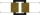The primary coil of the transformer has 1100 turns and is connected to a voltage of 220 V. How many turns does the secondary coil have when it has a voltage of 55 V? Determine the transformation ratio and decide what the transformation is.
• Resistor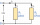Resistor 1 with a resistance of 100 Ohms and resistor 2 with a resistance of 400 Ohms are connected side by side in the circuit. There is a voltage of 80V between the resistor terminals. a) Draw a circuit diagram and write the entered quantities in it b)
• Mains voltageHow much electric current flows through the appliance with a resistance of 40kΩ, which is connected to the mains voltage (230 V)?
• Filament of bulbThe filament of bulb has a 1 ohm resistivity and is connected to a voltage 220 V. How much electric charge will pass through the fiber when the electric current passes for 10 seconds?
• Coils of transformerThe primary coil of the transformer has 400 turns, a current of 1.5 A passes through it and is connected to a voltage of 220 V. For the secondary coil, find the voltage, current, and a number of turns if the transformation ratio k = 0.1.
• Resistors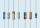Two resistors connected in series give the resulting resistance 245Ω and 60Ω in parallel. Determine the resistance of these resistors.
• Lowest voltage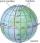Three resistors with resistors R1 = 10 kΩ, R2 = 20 kΩ, R3 = 30 kΩ are connected in series and an external voltage U = 30 V is connected to them. On which resistor is the lowest voltage?
• The outputThe output voltage of the transformer is 880 V. The secondary coil has 1200 turns. Find the voltage to which the primary coil is connected and how many turns it has if a current of 1 A flows through it. Transformation ratio k = 4. What current flows throu
• Coils of transformerThe primary coil of the transformer has 1100 turns and is connected to a voltage of 220V. How many turns does the secondary coil have when the voltage on it is 55 V? Find the transformation ratio and decide what kind of transformation.
• Athletic competitionIn a 400 meter athletic competition, a participant covers the distance as given below. find the average speed? first 80 meters 10 m/s next 240 meters 7.5 m/s last 80 meters 10 m/s
• Voltmeter range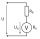We have a voltmeter which in the original set measures voltage to 10V. Calculate the size of the ballast resistor for this voltmeter, if we want to measure the voltage up to 50V. Voltmeter's internal resistance is 2 kiloohm/Volt.
• Current in the conductorCalculate the current in the conductor (in mA) if it is connected to a 4.5 V voltage source and its resistance is 20 (ohm).
• Coils of transformerWhat voltage is generated on the secondary coil of the transformer, which has 1500 turns, if the primary coil is connected to a voltage of 120 V and has 500 turns? What is the transformation ratio and what is the transformation about?